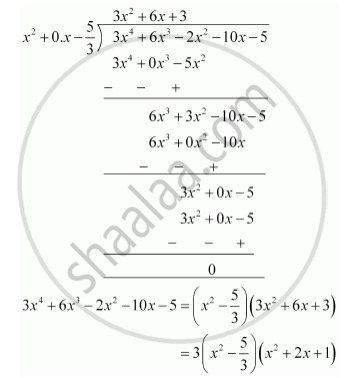# Obtain all other zeroes of 3x^4 + 6x^3 – 2x^2 – 10x – 5, if two of its zeroes are sqrt(5/3) and - sqrt(5/3) - Mathematics

Obtain all other zeroes of 3x4 + 6x3 – 2x2 – 10x – 5, if two of its zeroes are sqrt(5/3) and - sqrt(5/3)

#### Solution

p(x) = 3x4 + 6x3 – 2x2 – 10x – 5

Since the two zeroes are sqrt(5/3) and – sqrt(5/3).

∴ (x-sqrt(5/3))(x+sqrt(5/3)) = (x^2-5/3) is factor of 3x4+6x3-2x2-10x-5

Therfore, we divide the given polynomial by x^2-5/3we factorize x2+2x+1

=(x+1)2

Therefore, its zero is given by x+1=0

x = -1

As it has the term (x + 1)2 , therefore, there will be 2 zeroes at x = – 1.

Hence, the zeroes of the given polynomial are sqrt(5/3) and – sqrt(5/3) , – 1 and – 1

Concept: Division Algorithm for Polynomials
Is there an error in this question or solution?
Chapter 2: Polynomials - Exercise 2.3 [Page 36]

#### APPEARS IN

NCERT Class 10 Maths
Chapter 2 Polynomials
Exercise 2.3 | Q 3 | Page 36

Share